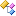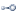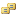﻿ Accord.Fuzzy Namespace# Accord.Fuzzy NamespaceClasses
ClassDescriptionCentroidDefuzzifier
This class implements the centroid defuzzification method.Clause
This class represents a fuzzy clause, a linguistic expression of the type "Variable IS Value".Database
The class represents a fuzzy database, a set of linguistic variables used in a Fuzzy Inference System.FuzzyOutput
The class represents the output of a Fuzzy Inference System.FuzzyOutputOutputConstraint
Inner class to store the pair fuzzy label / firing strength of a fuzzy output.FuzzySet
The class represents a fuzzy set.InferenceSystem
This class represents a Fuzzy Inference System.LinguisticVariable
The class represents a linguistic variable.MaximumCoNorm
Maximum CoNorm, used to calculate the linguistic value of a OR operation.MinimumNorm
Minimum Norm, used to calculate the linguistic value of a AND operation.NotOperator
NOT operator, used to calculate the complement of a fuzzy set.PiecewiseLinearFunction
Membership function composed by several connected linear functions.ProductNorm
Product Norm, used to calculate the linguistic value of a AND operation.Rule
This class represents a Fuzzy Rule, a linguistic expression representing some behavioral aspect of a Fuzzy Inference System.Rulebase
The class represents a fuzzy rulebase, a set of fuzzy rules used in a Fuzzy Inference System.SingletonFunction
Membership function used in fuzzy singletons: fuzzy sets that have just one point with membership value 1.TrapezoidalFunction
Membership function in the shape of a trapezoid. Can be a half trapzoid if the left or the right side is missing.Interfaces
InterfaceDescriptionICoNorm
Interface with the common methods of a Fuzzy CoNorm.IDefuzzifier
Interface which specifies set of methods required to be implemented by all defuzzification methods that can be used in Fuzzy Inference Systems.IMembershipFunction
Interface which specifies set of methods required to be implemented by all membership functions.INorm
Interface with the common methods of a Fuzzy Norm.IUnaryOperator
Interface with the common methods of Fuzzy Unary Operator.Enumerations
EnumerationDescriptionTrapezoidalFunctionEdgeType
Enumeration used to create trapezoidal membership functions with half trapezoids.